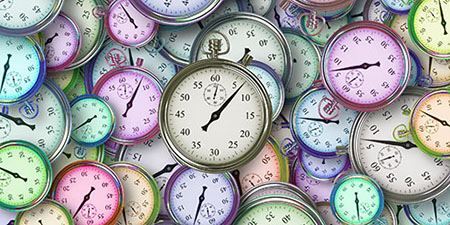# Minute (min) to Second (s)

Time units ﹣ Converter Minute to Second

Here you can convert the Time unit Minute into the unit Second and vice versa you can convert Second into Minute. By clicking the "Swap units" icon, you will always obtain the desired conversion in the calculation result, i.e., min to s or s to min. With the following calculator you can also calculate any other Time unit.

The minute (min) is not part of the SI International System of Units, but it is approved for use with the SI. The minute is derived from the base unit second. This makes it a legal unit of measurement.

Therefore, 1 minute equals 60 seconds, or 1 second equals 0.0166667 minutes.

After the day had already been divided into 24 units in ancient Egypt, Greek astronomers began 2000 years to divide each of these 24 units into 60 units using the Babylonian sexagesimal system. These 60 units were known as the "diminished part" (Latin: pars minuta), and this led to the term called “minute”. The “minutes” were again subdivided into 60 subunits (i.e., a "second diminished part" (Latin: pars minuta secunda), which in turn is termed "second". Therefore, the minute was defined astronomically as one-60th of an hour, and thus as one-60th of one-24th of a day.

It is now known that the earth's rotation slightly slows down, so the astronomical days always become minimally longer. The basic unit of time (the second) is now measured by atomic clocks, which are more accurate than the astronomical day. Therefore, a leap second must be added every 2 to 5 years in order to synchronise the atomic clocks with the earth's rotation again. As a result, a calendar minute then lasts 61 seconds.

The second (s) is the base unit of time in the International System of Units (SI). Therefore, the second is the physical unit of time measurement.

All other units of time are derived from the second (e.g., 1 minute = 60 seconds, 1 second = 1,000 milliseconds).

Until the middle of the 20th century, the second was defined as the sixtieth part of a minute of the day divided into 24 hours of 60 minutes. Since the rotation of the earth was assumed to be uniform, the length of a day and that of a second always appeared to be the same. It was not until 1934 that the long-held assumption that the Earth's rotation is not uniform was proven. As a result of magma displacements beneath the Earth's mantle and tidal friction, the Earth's rotation gradually slows down and thus the astronomical length of the day becomes longer. It turned out that the continuously improved quartz clocks provided a more consistent measure of time than the Earth's rotation. For this reason, a leap second is now added every 2 to 5 years to synchronise all clocks with the day, which always becomes a few fractions of a second longer.

Since 1967, the second has been defined as 9,192,631,770 times the period of a microwave, which resonates with a selected transition between two energy levels in the cesium atomic resonance. Therefore, it is called the atomic second Based on this definition. Atomic clocks are based on the exact measurement of this transition, and thus supersede quartz clocks in terms of accuracy.

## Basis for conversion Minute (min) to Second (s) and vice versa

The abbreviation for the "Time unit Minute" is min. The abbreviation for the "Time unit Second" is s.

## Formula for the conversion of Minute (min) to Second (s) and vice versa

The calculation from Minute to Second shall be made using the following conversion formula:

Conversion formula Minute to Second

Determine the number of Second from Minute

Minute × 60

## Formula for the conversion of Second (s) to Minute (min)

The calculation from Second to Minute shall be made using the following conversion formula:

Conversion formula Second to Minute

Determine the number of Minute from Second

Second × 0.016666666666667

## Overview table : How many Minute are in a Second ?

Minute min ⇒ Second s
0.01 min  are  0.60000 s
0.02 min  are  1.20000 s
0.03 min  are  1.80000 s
0.04 min  are  2.40000 s
0.05 min  are  3 s
0.06 min  are  3.60000 s
0.07 min  are  4.20000 s
0.08 min  are  4.80000 s
0.09 min  are  5.40000 s
0.10 min  are  6 s
0.20 min  are  12 s
0.30 min  are  18 s
0.40 min  are  24 s
0.50 min  are  30 s
0.60 min  are  36 s
0.70 min  are  42 s
0.80 min  are  48 s
0.90 min  are  54 s
1 min  corresponds to  60 s
2 min  are  120 s
3 min  are  180 s
4 min  are  240 s
5 min  are  300 s
6 min  are  360 s
7 min  are  420 s
8 min  are  480 s
9 min  are  540 s
10 min  are  600 s
20 min  are  1 200 s
30 min  are  1 800 s
40 min  are  2 400 s
50 min  are  3 000 s
60 min  are  3 600 s
70 min  are  4 200 s
80 min  are  4 800 s
90 min  are  5 400 s
100 min  are  6 000 s
200 min  are  12 000 s
300 min  are  18 000 s
400 min  are  24 000 s
500 min  are  30 000 s
600 min  are  36 000 s
700 min  are  42 000 s
800 min  are  48 000 s
900 min  are  54 000 s
1 000 min  are  60 000 s

## Overview table : How many Second are in a Minute ?

Second s ⇒ Minute min
0.01 s  are  0.00016 min
0.02 s  are  0.00033 min
0.03 s  are  0.00050 min
0.04 s  are  0.00066 min
0.05 s  are  0.00083 min
0.06 s  are  0.00100 min
0.07 s  are  0.00116 min
0.08 s  are  0.00133 min
0.09 s  are  0.00150 min
0.10 s  are  0.00166 min
0.20 s  are  0.00333 min
0.30 s  are  0.00500 min
0.40 s  are  0.00666 min
0.50 s  are  0.00833 min
0.60 s  are  0.01000 min
0.70 s  are  0.01166 min
0.80 s  are  0.01333 min
0.90 s  are  0.01500 min
1 s  corresponds to  0.01666 min
2 s  are  0.03333 min
3 s  are  0.05000 min
4 s  are  0.06666 min
5 s  are  0.08333 min
6 s  are  0.10000 min
7 s  are  0.11666 min
8 s  are  0.13333 min
9 s  are  0.15000 min
10 s  are  0.16666 min
20 s  are  0.33333 min
30 s  are  0.50000 min
40 s  are  0.66666 min
50 s  are  0.83333 min
60 s  are  1 min
70 s  are  1.16666 min
80 s  are  1.33333 min
90 s  are  1.50000 min
100 s  are  1.66666 min
200 s  are  3.33333 min
300 s  are  5 min
400 s  are  6.66666 min
500 s  are  8.33333 min
600 s  are  10 min
700 s  are  11.66666 min
800 s  are  13.33333 min
900 s  are  15 min
1 000 s  are  16.66666 min